Orbits in Strongly Curved Spacetime

Your browser does not support HTML5 canvas. You may be able to use the Java version of this page.

Introduction

The display above shows, from three different physical perspectives, the orbit of a low-mass test particle, the small red circle, around a non-rotating black hole (represented by a grey circle in the panel at the right, where the radius of the circle is the black hole's gravitational radius, or event horizon. Kepler's laws of planetary motion, grounded in Newton's theory of gravity, state that the orbit of a test particle around a massive object is an ellipse with one focus at the centre of the massive object. But when gravitational fields are strong, as is the case for collapsed objects like neutron stars and black holes, Newton's theory is inaccurate; calculations must be done using Einstein's theory of General Relativity.

In Newtonian gravitation, an orbit is always an ellipse. As the gravitating body becomes more massive and the test particle orbits it more closely, the speed of the particle in its orbit increases without bound, always balancing the gravitational force. For a black hole, Newton's theory predicts orbital velocities greater than the speed of light, but according to Einstein's Special Theory of Relativity, no material object can achieve or exceed the speed of light. In strong gravitational fields, General Relativity predicts orbits drastically different from the ellipses of Kepler's laws. This page allows you to explore them.

The Orbit Plot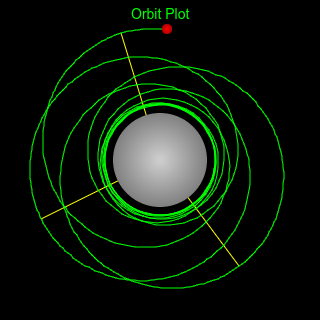The panel at the right shows the test mass orbiting the black hole, viewed perpendicular to the plane of its orbit. The path of the orbit is traced by the green line. After a large number of orbits the display will get cluttered; just click the mouse anywhere in the right panel to erase the path and start over. When the test mass reaches its greatest distance from the black hole, a yellow line is plotted from the centre of the black hole to that point, the apastron of the orbit. In Newtonian gravity, the apastron remains fixed in space. The effects of General Relativity cause it to precess. You can see the degree of precession in the displacement of successive yellow lines (precession can be more than 360°; the yellow line only shows precession modulo one revolution).

The Gravitational Effective-Potential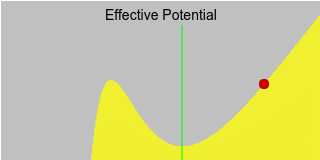The panels at the left display the orbit in two more abstract ways. The Effective Potential plot at the top shows the position of the test mass on the gravitational energy curve as it orbits in and out. The summit on the left side of the curve is unique to General Relativity—in Newtonian gravitation the curve rises without bound as the radius decreases, approaching infinity at zero. In Einstein's theory, the inability of the particle to orbit at or above the speed of light creates a “pit in the potential” near the black hole. As the test mass approaches this summit, falling in from larger radii with greater and greater velocity, it will linger near the energy peak for an increasingly long time, while its continued angular motion will result in more and more precession. If the particle passes the energy peak and continues to lesser radii, toward the left, its fate is sealed—it will fall into the black hole and be captured.

The Gravity Well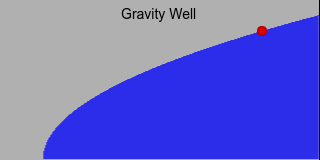Spacetime around an isolated spherical non-rotating uncharged gravitating body is described by Schwarzschild Geometry, in which spacetime can be thought of as being bent by the presence of mass. This creates a gravity well which extends to the surface of the body or, in the case of a black hole, to oblivion. The gravity well has the shape of a four-dimensional paraboloid of revolution, symmetrical about the central mass. Since few Web browsers are presently equipped with four-dimensional display capability, I've presented a two-dimensional slice through the gravity well in the panel at the bottom left. Like the energy plot above, the left side of the panel represents the centre of the black hole and the radius increases to the right. Notice that the test mass radius moves in lockstep on the two charts, as the radius varies on the orbit plot to their right.

The gravity well of a Schwarzschild black hole has a throat at a radius determined solely by its mass—that is the location of the hole's event horizon; any matter or energy which crosses the horizon is captured. The throat is the leftmost point on the gravity well curve, where the slope of the paraboloidal geometry becomes infinite (vertical). With sufficient angular momentum, a particle can approach the event horizon as closely as it wishes (assuming it is small enough so it isn't torn apart by tidal forces), but it can never cross the event horizon and return.

Hands On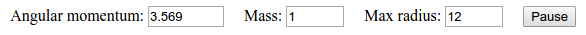By clicking in the various windows and changing values in the controls at the bottom of the window you can explore different scenarios. To pause the simulation, press the Pause button at the right; pressing it again resumes the simulation. Click anywhere in the orbit plot at the right to clear the orbital trail and apastron markers when the screen becomes too cluttered. You can re-launch the test particle at any given radius from the black hole (with the same angular momentum) by clicking at the desired radius in either the Effective Potential or Gravity Well windows. The green line in the Effective Potential plot indicates the energy minimum at which a stable circular orbit exists for a particle of the given angular momentum.

The angular momentum is specified by the box at left in terms of the angular momentum per unit mass of the black hole, all in geometric units—all of this is explained in detail below. What's important to note is that for orbits like those of planets in the Solar System, this number is huge; only in strong gravitational fields does it approach small values. If the angular momentum is smaller than a critical value (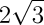, about 3.464 for a black hole of mass 1, measured in the same units), no stable orbits exist; the particle lacks the angular momentum to avoid being swallowed. When you enter a value smaller than this, notice how the trough in the energy curve and the green line marking the stable circular orbit disappear. Regardless of the radius, any particle you launch is doomed to fall into the hole.

The Mass box allows you to change the mass of the black hole, increasing the radius of its event horizon. Since the shape of the orbit is determined by the ratio of the angular momentum to the mass, it's just as easy to leave the mass as 1 and change the angular momentum. You can change the scale of all the panels by entering a new value for the maximum radius; this value becomes the rightmost point in the effective potential and gravity well plots and the distance from the centre of the black hole to the edge of the orbit plot. When you change the angular momentum or mass, the radius scale is automatically adjusted so the stable circular orbit (if any) is on screen.

Kepler, Newton, and Beyond

In the early 17th century, after years of tedious calculation and false starts, Johannes Kepler published his three laws of planetary motion:

• First law (1605): A planet's orbit about the Sun is an ellipse, with the Sun at one focus.
• Second law (1604): A line from the Sun to a planet sweeps out equal areas in equal times.
• Third law (1618): The square of the orbital period of a planet is proportional to the cube of the major axis of the orbit.

Kepler's discoveries about the behaviour of planets in their orbits played an essential rôle in Isaac Newton's formulation of the law of universal gravitation in 1687. Newton's theory showed the celestial bodies were governed by the same laws as objects on Earth. The philosophical implications of this played as key a part in the Enlightenment as did the theory itself in the subsequent development of physics and astronomy.

While Kepler's laws applied only to the Sun and planets, Newton's universal theory allowed one to calculate the gravitational force and motion of any bodies whatsoever. To be sure, when many bodies were involved and great accuracy was required, the calculations were horrifically complicated and tedious—so much so that those reared in the computer age may find it difficult to imagine embarking upon them armed with nothing but a table of logarithms, pencil and paper, and the human mind. But performed they were, with ever greater precision as astronomers made increasingly accurate observations. And those observations agreed perfectly with the predictions of Newton's theory.

Well,… almost perfectly. After painstaking observations of the planets and extensive calculation, astronomer Simon Newcomb concluded in 1898 that the orbit of Mercury was precessing 43 arc-seconds per century more than could be explained by the influence of the other planets. This is a tiny discrepancy, but further observations and calculations confirmed Newcomb's—the discrepancy was real. Some suggested a still undiscovered planet closer to the Sun than Mercury (and went so far as to name it, sight unseen, “Vulcan”), but no such planet was ever found, nor any other plausible explanation advanced. For nearly twenty years Mercury's precession or “perihelion advance” remained one of those nagging anomalies in the body of scientific data that's trying to tell us something, if only we knew what.

In 1915, Albert Einstein's General Theory of Relativity extended Newtonian gravitation theory, revealing previously unanticipated subtleties of nature. And Einstein's theory explained the perihelion advance of Mercury. That tiny discrepancy in the orbit of Mercury was actually the first evidence for what lay beyond Newtonian gravitation, the first step down a road that would lead to understanding black holes, gravitational radiation, and the source of inertia, which remains a fertile ground for theoretical and experimental physics a century thereafter.

Geometric Units

The force of gravity is so weak compared to the electromagnetic force which binds objects on the human scale, and the speed of light so great compared to velocities with which we're familiar, that calculating the effects of general relativity, which involve both the gravitational force and the speed of light, using conventional units like grams, centimetres, and seconds usually results in enormous or minuscule quantities cumbersome to manipulate and difficult to understand intuitively.

If we're interested in the domain where general relativistic effects are substantial, we're better off calculating with units scaled to the problem. A particularly convenient and elegant choice is the system of geometric units, obtained by setting Newton's gravitational constant G, the speed of light c, and Boltzmann's constant k all equal to 1. We can then express any of the following units as a length in centimetres by multiplying by the following conversion factors.

Quantity Unit cm Equivalent
Time second 2.997930×1010 cm/sec
Mass gram 0.7425×10−28 cm/g
Energy erg 0.826×10−49 cm/erg
Electric charge e 1.381×10−28 cm/e
Temperature ° Kelvin 1.140×10−65 cm/°K

The enormous exponents make it evident that these units are far removed from our everyday experience. It would be absurd to tell somebody, “I'll call you back in 1.08×1014 centimetres”, but it is a perfectly valid way of saying “one hour”. The discussion that follows uses geometric units throughout, allowing us to treat mass, time, length, and energy without conversion factors. To express a value calculated in geometric units back to conventional units, just divide by the value in the table above.

The Gravitational Effective-PotentialThe gravitational effective-potential for a test particle orbiting in a Schwarzschild geometry is: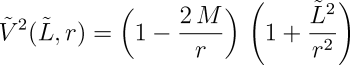whereis the angular momentum per unit rest mass expressed in geometric units, M is the mass of the gravitating body, and r is the radius of the test particle from the centre of the body.

The radius of a particle from the centre of attraction evolves in proper time τ (time measured by a clock moving along with the particle) according to: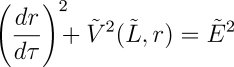where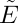is the potential energy of the test mass at infinity per rest mass.

Angular motion about the centre of attraction is then:while time, as measured by a distant observer advances according to: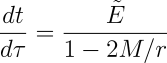and can be seen to slow down as the event horizon at the gravitational radius is approached. At the gravitational radius of 2M time, as measured from far away, stops entirely so the particle never seems to reach the event horizon. Proper time on the particle continues to advance unabated; an observer on-board sails through the event horizon without a bump and continues toward the doom which awaits at the central singularity.

Circular Orbits

Circular orbits are possible at maxima and minima of the effective-potential. Orbits at minima are stable, since a small displacement increases the energy and thus creates a restoring force in the opposite direction. Orbits at maxima are unstable; the slightest displacement causes the particle to either be sucked into the black hole or enter a highly elliptical orbit around it.

To find the radius of possible circular orbits, differentiate the gravitational effective-potential with respect to the radius r: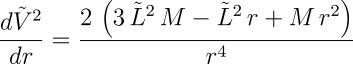The minima and maxima of a function are at the zero crossings of its derivative, so a little algebra gives the radii of possible circular orbits as:The larger of these solutions is the stable circular orbit, while the smaller is the unstable orbit at the maximum. For a black hole, this radius will be outside the gravitational radius at 2M, while for any other object the radius will be less than the diameter of the body, indicating no such orbit exists. If the angular momentum L² is less than 12M², no stable orbit exists; the object will impact the surface or, in the case of a black hole, fall past the event horizon and be swallowed.

References

 Click on titles to order books on-line from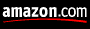Gallmeier, Jonathan, Mark Loewe, and Donald W. Olson. “Precession and the Pulsar.” Sky & Telescope (September 1995): 86–88.
A BASIC program which plots orbital paths in Schwarzschild geometry. The program uses different parameters to describe the orbit than those used here, and the program does not simulate orbits which result in capture or escape. This program can be downloaded from the Sky & Telescope Web site.
Misner, Charles W., Kip S. Thorne, and John Archibald Wheeler. Gravitation. San Francisco: W. H. Freeman, 1973. ISBN 978-0-7167-0334-1.
Chapter 25 thoroughly covers all aspects of motion in Schwarzschild geometry, both for test particles with mass and massless particles such as photons.
Wheeler, John Archibald. A Journey into Gravity and Spacetime. New York: W. H. Freeman, 1990. ISBN 978-0-7167-5016-1.
This book, part of the Scientific American Library series (but available separately), devotes chapter 10 to a less technical discussion of orbits in Schwarzschild spacetime. The “energy hill” on page 173 and the orbits plotted on page 176 provided the inspiration for this page.

by John Walker
February 1997
Update: December 1998
Update: June 2008
Update: January 2017